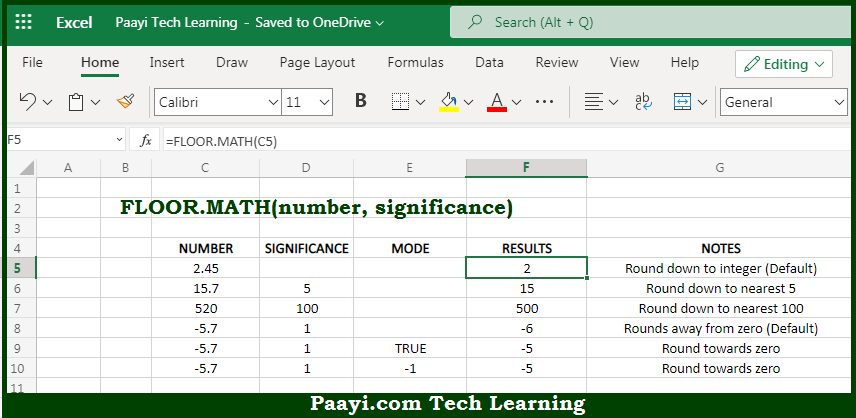# Learn How to Use Microsoft Excel FLOOR.MATH Function

Written by | 0 Comments | 605 Views

In this article, you will learn how to use the Microsoft Excel FLOOR.MATH function and its prime function in Microsoft Excel. You will also get to know the Microsoft Excel FLOOR.MATH function returns value and syntax with the help of some examples.

Microsoft Excel FLOOR.MATH Function

The main function of Microsoft Excel FLOOR.MATH function is to round down the number to the nearest multiple. So, with the help of FLOOR.MATH function, you can able to round up the given number, to the nearest specified multiple. It should be noted that, the FLOOR.MATH is different as compared with FLOOR function, as FLOOR.MATH defaults to a multiple of 1 and offers complete support for rounding negative numbers. So, with the help of Microsoft Excel FLOOR.MATH function you can easily round down the number to the nearest multiple.

Return Value of FLOOR.MATH Function

The return value will be the round-down number.

Syntax of FLOOR.MATH Function

=FLOOR.MATH(number, [significance], [mode])

Where the arguments:

• number: This is the number of which you want to round down.
• significance: This is multiple to use while rounding down the number, the default is 1 (optional).
• mode: It is used to rounding off the negative numbers towards or away from zero, default is 0 (optional).

## How to Use Microsoft Excel FLOOR.MATH Function?So we know that, Microsoft Excel FLOOR.MATH function you can able to round up the given number, to the nearest specified multiple. Positive numbers with decimal values are rounded down to the nearest integer, for example,  5.7 is rounded down to 5, while negative numbers with decimal values are rounded away from zero for example, -6.8 is rounded to -7. It should be noted that, the FLOOR.MATH is different as compared with FLOOR function, as FLOOR.MATH defaults to a multiple of 1 and offers complete support for rounding negative numbers. So, with the help of Microsoft Excel FLOOR.MATH function you can easily round down the number to the nearest multiple.